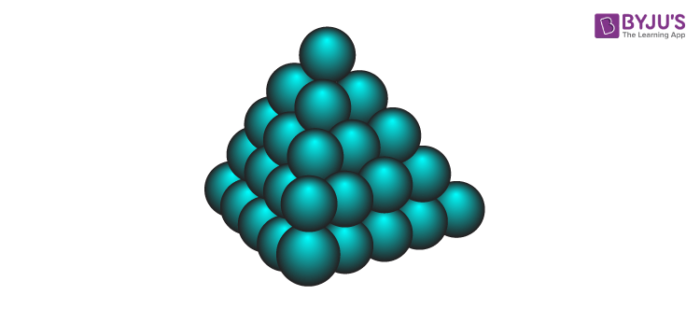Checkout JEE MAINS 2022 Question Paper Analysis : Checkout JEE MAINS 2022 Question Paper Analysis :

# Enthalpy: Definition and Derivation

You already know that heat absorbed or released by a body is related to its internal energy. The heat absorbed is equal to the change in the internal energy. This is given by ∆U = qV. But this equation holds good only at a constant volume. However, when heat is absorbed or released by a body, there is a change in its volume. The alternate solution is to carry out the reaction at a constant pressure – in flasks and test tubes. Hence, there is a need for another state function which defines the change in internal energy at a constant pressure. The concept of enthalpy comes into picture here.#### Enthalpy

At a constant pressure, the equation for change in internal energy, ∆U = q + w can be written as

∆U = qP – p∆V

Where qP represents the heat absorbed by the system at a constant pressure and – p∆V is the expansion work done due to the heat absorbed by the system.

We can write the above equation in terms of initial and final states of the system as:

UF – UI = qP –p(VF – VI)

Or qP = (UF + pVF) – (UI + pVI)

Enthalpy H can be given by H = U + PV. Substituting it in the above equation, we get:

qP = HF – HI = ∆H

Hence, change in enthalpy ∆H = qP, which is the heat absorbed by the system at a constant pressure.

At constant pressure, we can also write,

∆H = ∆U + p∆V

Some pointers to be kept in mind:

• In exothermic reactions, heat from the system is lost to the surrounding. For such reactions, ∆H is negative.
• In endothermic reactions, heat is absorbed by the system from the surroundings. For such reactions, ∆H is positive.